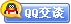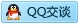##用户名 Email 自动登录 找回密码 密码 会员注册
 VIP会员，3年作业免费下 ！ 奥鹏作业，奥鹏毕业论文检测 新手作业下载教程，充值问题 没有找到答案，请在此处留言！ 2019年12月最新全国统考资料 投诉建议，加盟合作！奥鹏课程积分软件(ver:3.1)

# 吉大19年11月清考《高等数学（理专）》作业考核试题发表于 2019-11-5 17:34:30 | 显示全部楼层 |阅读模式吉大19年11月清考《高等数学（理专）》作业考核试题-0001 试卷总分:100  得分:100 一、单选题 (共 15 道试题,共 60 分) 1.函数y=e^(cx)+1是微分方程yy"=(y')^2+y"的（） A.通解 B.特解 C.不是解 D.是解，但既不是通解，也不是特解 答案2.函数y=|sinx|在x=0处( ) A.无定义 B.有定义，但不连续 C.连续 D.无定义，但连续 答案:C 3.下列函数中 （ ）是奇函数 A.xsinx B.x+cosx C.x+sinx D.|x|+cosx 答案:C 4.设f(x)=x(x-1)(x-2)(x-3),则f’(0)=( ) A.-6 B.-2 C.3 D.-3 答案: 5.已知函数y= 2cos3x-5e2x, 则x=0时的微分dy=（） A.10 B.10dx C.-10 D.-10dx 答案: 6.集合A={±2，±3，±4，±5，±6}表示 A.A是由绝对值小于等于6的全体整数组成的集合 B.A是由绝对值大于等于2，小于等于6的全体整数组成的集合 C.A是由全体整数组成的集合 D.A是由绝对值大于2，小于6的整数组成的集合 答案: 7.微分方程y'+y=x+1的一个特解是（） A.x+y=0 B.x-y=0 C.x+y=1 D.x-y=1 答案: 8.对于函数f(x)=[(x^2-1)(x^2-4)]^(2/3),下列能满足罗尔定理条件的区间是（） A.[0,√5] B.[-1,1] C.[-2,1] D.[-1,2] 答案: 9.求极限lim_{x->0} tanx/x = ( ) A.0 B.1 C.2 D.1/e 答案: 10.求极限lim_{n->无穷} n^2/(2n^2+1) = ( ) A.0 B.1 C.1/2 D.3 答案: 11.函数f(x)=(x^2-x-2)|x^3-x|的不可导点的个数为（） A.0 B.1 C.2 D.3 答案: 12.微分方程ydx+xdy=0的通解是() A.xy=C B.xy=0 C.x+y=C D.x-y=0 答案: 13.已知f(x)的一个原函数是e^(-x),则∫xf'(x)dx等于（） A.xe^(-x)+e^(-x)+C B.xe^(-x)-e^(-x)+C C.-xe^(-x)-e^(-x)+C D.-xe^(-x)+e^(-x)+C 答案: 14.集合B是由能被3除尽的全部整数组成的，则B可表示成 A.{3，6，…，3n} B.{±3，±6，…，±3n} C.{0，±3，±6，…，±3n…} D.{0，±3，±6，…±3n} 答案: 15.下列结论正确的是（） A.若|f(x)|在x=a点处连续，则f(x)在x=a点也必处连续 B.若[f(x)]^2在x=a点处连续，则f(x)在x=a点也必处连续 C.若[f(x)]^3在x=a点处连续，则f(x)在x=a点也必处连续 D.若f(x)在x=a点处连续，则1/f(x)在x=a点也必处连续 答案: 二、判断题 (共 10 道试题,共 40 分) 16.函数y=sinx没有拐点存在。（） 答案: 17.一元函数可导的充要条件是左右导数都存在且相等。 答案: 18.函数y=sinx没有拐点存在。（） 答案: 19.复合函数对自变量的导数，等于函数对中间变量的导数乘以中间变量对自变量的导数。 答案: 20.闭区间上连续函数在该区间上可积。 答案: 21.一元函数可导必连续，连续必可导。 答案: 22.如果f(x)在区间[a,b]上是单调有界函数，则f(x)在[a,b]上可积 答案: 23.一元函数可导的充要条件是左右导数都存在且相等。 答案: 24.两个无穷大量的和仍是无穷大。 答案: 25.所有初等函数及其复合得到的函数的导数如果存在，也是初等函数及其复合。（ ） 答案:

### 本帖子中包含更多资源

x
B Color Image Link Quote Code Smilies |上传客服一客服二客服三客服四微信客服扫一扫